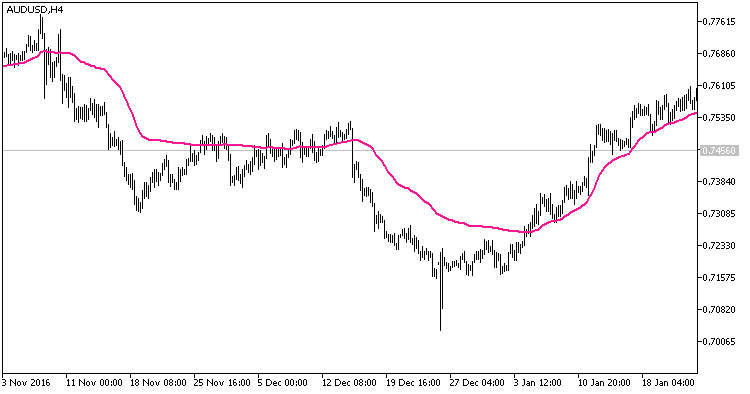# EMAVFS – indicator for MetaTrader 5

• A+
category：MT5 INDICATORS

Real author: Stanislav Bulashev

Exponential moving average with a variable smoothing factor.

```//+-----------------------------------+
//|   INDICATOR INPUT PARAMETERS      |
//+-----------------------------------+
input double Wmin=0;                      // minimum sensitivity
input double Wmax=5;                      // maximum sensitivity
input double Efactor=1.01;                // factor E (change the value near one for the tenth and hundredth shares)
input double Afactor=1.001;               // degree A (change the value near one for the tenth and hundredth shares)
input Applied_price_ IPC=PRICE_CLOSE_;    // price constant
input int Shift=0;                        // horizontal shift of the indicator in bars
input int PriceShift=0;                   // vertical shift of the indicator in points
//+-----------------------------------+
```

This indicator is compiled as both an MQL5 indicator and an MQL4 indicator, for which the extension of the indicator code should be changed for mq4, place the code in <terminal_data_directory MetaTrader4>\MQL4\Indicators, and compile it using the relevant code editor.Fig.1. Indicator EMAVFS